Python Code GolfCurrent challenge:
"Determine if a sentence is a palindrome."

Current shortest code:
70 bytes

Current contest ends:
1579996800, F jS, g:i A
Give it a try
So you want to make a game? You have choices. But basically, your choices are determined by three constraints:
• What sort of language do you want to program in?
• What sort of platform do you want to deploy your game to?
• What sort of game are you making?

Most of the time you can answer each of these questions independently and find a perfect language/framework that fits your needs. Other times, you are constrained. For example, there aren't many HTML5 frameworks that allow you to write a high-performance 3D game.

For PyGame, I am going to assume you gave the following answers to the previous 3 questions:
• You want to program in Python. Also, you already know Python. Teaching Python from scratch is not covered in this tutorial.
• You want to create a client app that can potentially be wrapped in a standalone executable. You don't care about playing it in a browser or on a mobile device.
• The game you want to create is graphical, but not 3D.

If this sounds like your situation, continue.

## Setting Up Python

I recommend Python 2.7 for various reasons I won't go in to here. If you're on windows, just get the Windows Installer. Installation is simple. Just hit Next Next Next Next Finish. Defaults are fine.

Be sure to download the version that corresponds to the version of Python you downloaded just now (and the version that corresponds to your operating system, of course). If you downloaded Python 2.7 like I suggested, you would download the pygame-1.9.2a0.win32-py2.7.msi if you're on Windows. If you're not on Windows, then you probably know what you're doing anyway. Installation of PyGame is equally as simple as Python. Just go with the defaults.

## The Anatomy of a PyGame Game

The following is the simplest barebones app that can be made using the PyGame pipeline:
import pygame

pygame.init()
screen = pygame.display.set_mode((400300))
done = False

while not done:
for event in pygame.event.get():
if event.type == pygame.QUIT:
done = True

pygame.display.flip()

import pygame - this is of course needed to access the PyGame framework.
pygame.init() - This kicks things off. It initializes all the modules required for PyGame.
pygame.display.set_mode((width, height)) - This will launch a window of the desired size. The return value is a Surface object which is the object you will perform graphical operations on. This will be discussed later.
pygame.event.get() - this empties the event queue. If you do not call this, the windows messages will start to pile up and your game will become unresponsive in the opinion of the operating system.
pygame.QUIT - This is the event type that is fired when you click on the close button in the corner of the window.
pygame.display.flip() - PyGame is double-buffered. This swaps the buffers. All you need to know is that this call is required in order for any updates that you make to the game screen to become visible.

When you run this, you'll see: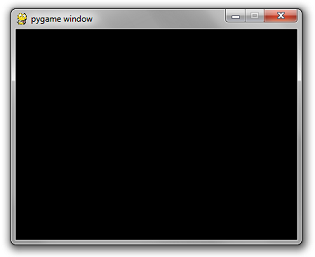Which is not amazing at all. Because it's a black box that does nothing.

## Drawing Something

pygame.draw.rect - As you can imagine, this will draw a rectangle. I takes in a few arguments, including the surface to draw on (I'll be drawing on the screen instance), the color, and the coordinates/dimensions of the rectangle.

# Add this somewhere after the event pumping and before the display.flip()
pygame.draw.rect(screen, (0128255), pygame.Rect(30306060))

The first argument is the surface instance to draw the rectangle to.
The second argument is the (red, green, blue) tuple that represents the color to draw with.
The third argument is a pygame.Rect instance. The arguments for this constructor are the x and y coordintaes of the top left corner, the width, and the height.

Amazing-er!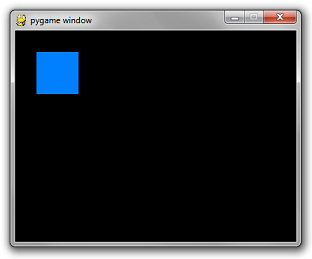...but not by much. Right now it may as well just be an image in a window. Next up: interactivity.

## Interactivity

The point of a game is to be interactive. Right now, the only thing you can interact with is the close button. Which isn't a very fun game. All user input events come through the event queue. Simply add more if statements to that for loop to add more interactivity.

Add the following code before the loop:
is_blue = True

Modify your rectangle code to pick a color conditionally:
if is_blue: color = (0128255)
else: color = (2551000)
pygame.draw.rect(screen, color, pygame.Rect(30306060))

Finally, the important bit. Add the following if statement to your for loop in the same sequence as the other if statement in there...
if event.type == pygame.KEYDOWN and event.key == pygame.K_SPACE:
is_blue = not is_blue

Press space to change the box color.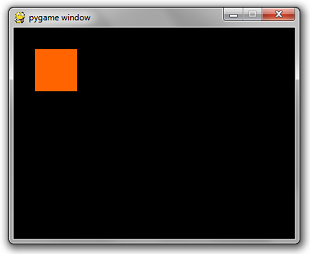As you can imagine, there is also a corresponding pygame.KEYUP event type and a pygame.K_%%%%% for almost every key on your keyboard. To see this list, go to a python terminal and use dir(pygame).
>>> import pygame
>>> filter(lambda x:'K_' in x, dir(pygame))
['K_0', 'K_1', 'K_2', 'K_3', 'K_4', 'K_5', 'K_6', 'K_7', 'K_8', 'K_9', 'K_AMPERS
AND', 'K_ASTERISK', 'K_AT', 'K_BACKQUOTE', 'K_BACKSLASH', 'K_BACKSPACE', 'K_BREA
K', 'K_CAPSLOCK', 'K_CARET', 'K_CLEAR', 'K_COLON', 'K_COMMA', 'K_DELETE', 'K_DOL
LAR', 'K_DOWN', 'K_END', 'K_EQUALS', 'K_ESCAPE', 'K_EURO', 'K_EXCLAIM', 'K_F1',
'K_F10', 'K_F11', 'K_F12', 'K_F13', 'K_F14', 'K_F15', 'K_F2', 'K_F3', 'K_F4', 'K
_F5', 'K_F6', 'K_F7', 'K_F8', 'K_F9', 'K_FIRST', 'K_GREATER', 'K_HASH', 'K_HELP'
, 'K_HOME', 'K_INSERT', 'K_KP0', 'K_KP1', 'K_KP2', 'K_KP3', 'K_KP4', 'K_KP5', 'K
_KP6', 'K_KP7', 'K_KP8', 'K_KP9', 'K_KP_DIVIDE', 'K_KP_ENTER', 'K_KP_EQUALS', 'K
_KP_MINUS', 'K_KP_MULTIPLY', 'K_KP_PERIOD', 'K_KP_PLUS', 'K_LALT', 'K_LAST', 'K_
LCTRL', 'K_LEFT', 'K_LEFTBRACKET', 'K_LEFTPAREN', 'K_LESS', 'K_LMETA', 'K_LSHIFT
', 'K_LSUPER', 'K_MENU', 'K_MINUS', 'K_MODE', 'K_NUMLOCK', 'K_PAGEDOWN', 'K_PAGE
UP', 'K_PAUSE', 'K_PERIOD', 'K_PLUS', 'K_POWER', 'K_PRINT', 'K_QUESTION', 'K_QUO
TE', 'K_QUOTEDBL', 'K_RALT', 'K_RCTRL', 'K_RETURN', 'K_RIGHT', 'K_RIGHTBRACKET',
'K_RIGHTPAREN', 'K_RMETA', 'K_RSHIFT', 'K_RSUPER', 'K_SCROLLOCK', 'K_SEMICOLON'
, 'K_SLASH', 'K_SPACE', 'K_SYSREQ', 'K_TAB', 'K_UNDERSCORE', 'K_UNKNOWN', 'K_UP'
, 'K_a', 'K_b', 'K_c', 'K_d', 'K_e', 'K_f', 'K_g', 'K_h', 'K_i', 'K_j', 'K_k', '
K_l', 'K_m', 'K_n', 'K_o', 'K_p', 'K_q', 'K_r', 'K_s', 'K_t', 'K_u', 'K_v', 'K_w
', 'K_x', 'K_y', 'K_z']
>>>

There are also mouse event types, but that will be covered later.

Our box is bored of switching from aquamarine to orangish red. He wants to move around.

There is an additional way to access key events. You can get the depression status of any key by calling pygame.key.get_pressed(). This returns a huge array filled with 1's and 0's. Mostly 0's.

When you check the integer value of any of the pygame.K_%%%%% constants, you'll notice it's a number.
>>> pygame.K_LEFTBRACKET
91
>>>

This value is not-so-coincidentally the index of the get_pressed() array that corresponds to that key. So if you want to see if the Up Arrow is pressed, the way to do that is:
up_pressed = pygame.get_pressed()[pygame.K_UP]

Simple as that.

This is useful for the sort of events that you want to do when the user holds down a button. For example, moving around a sprite when the user holds down any arrow keys.

Applying this concept to our current box game, this is what the code looks like now:
import pygame

pygame.init()
screen = pygame.display.set_mode((400300))
done = False
is_blue = True
x = 30
y = 30

while not done:
for event in pygame.event.get():
if event.type == pygame.QUIT:
done = True
if event.type == pygame.KEYDOWN and event.key == pygame.K_SPACE:
is_blue = not is_blue

pressed = pygame.key.get_pressed()
if pressed[pygame.K_UP]: y -= 3
if pressed[pygame.K_DOWN]: y += 3
if pressed[pygame.K_LEFT]: x -= 3
if pressed[pygame.K_RIGHT]: x += 3

if is_blue: color = (0128255)
else: color = (2551000)
pygame.draw.rect(screen, color, pygame.Rect(x, y, 6060))

pygame.display.flip()

## Hmm...that's not what I wanted...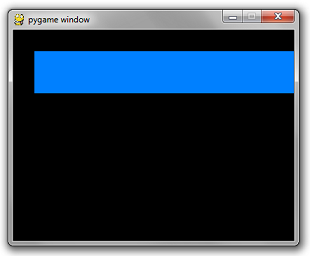Two things are wrong.
• Each time you draw a rectangle, the rectangle from the previous frames remains on the screen.
• It moves really really really fast.

For the first, you simply need to reset the screen to black before you draw the rectangle. There is a simple method on Surface called fill that does this. It takes in an rgb tuple.
screen.fill((000))

Secondly, the duration of each frame is as short as your super fancy computer can make it. The framerate needs to be throttled at a sane number such as 60 frames per second. Luckily, there is a simple class in pygame.time called Clock that does this for us. It has a method called tick which takes in a desired fps rate.

clock = pygame.time.Clock()

...
while not done:

...

# will block execution until 1/60 seconds have passed
# since the previous time clock.tick was called.
clock.tick(60)

Put it all together and you get:
import pygame

pygame.init()
screen = pygame.display.set_mode((400300))
done = False
is_blue = True
x = 30
y = 30

clock = pygame.time.Clock()

while not done:
for event in pygame.event.get():
if event.type == pygame.QUIT:
done = True
if event.type == pygame.KEYDOWN and event.key == pygame.K_SPACE:
is_blue = not is_blue

pressed = pygame.key.get_pressed()
if pressed[pygame.K_UP]: y -= 3
if pressed[pygame.K_DOWN]: y += 3
if pressed[pygame.K_LEFT]: x -= 3
if pressed[pygame.K_RIGHT]: x += 3

screen.fill((000))
if is_blue: color = (0128255)
else: color = (2551000)
pygame.draw.rect(screen, color, pygame.Rect(x, y, 6060))

pygame.display.flip()
clock.tick(60)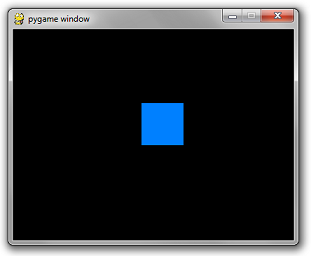You'll have to take my word on the fact that you can move it around.

That concludes this part of the tutorial.

Next up: Images.

Hey, there, Python folks. Hope you enjoyed the post. I just wanted to give a quick shout-out for a weekly Python code golf that I recently started up over on StringLabs.io. If you like Python puzzles please come check it out!